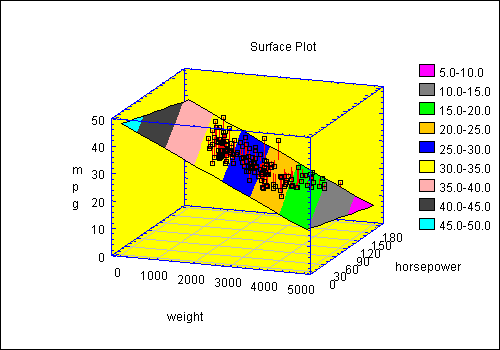# SurfacePlot Statbean®

Purpose: Creates 3D surface plots for a response surface.

3D Surface Chart DataSource: any.3D Surface Plot Software Read/Write Properties
 Name Type Description Possible Values Default Value addPoints boolean Whether to add points around the surface. true,false false contoursBy double The distance between the contours. Any double > 0. missing contoursFrom double The level at which the first contour should be plotted. Any double. missing (causes default scaling) contoursTo double The level at which the last contour should be plotted. Any double > contoursFrom. missing contourType String The type of contours to be plotted in the base. "Lines","Regions" "Regions" drawContoursBelow boolean Whether to draw contours in the base of the base. true,false false gridSize int The number of values along each dimension of the matrix which defines the surface. 2+ 21 horizontalViewAngle int Angle from which plot is viewed in degrees. Any integer. 20 surfaceGrid double[gridSize][gridSize] An array containing the height of the surface. Any double. none surfaceType String The type of surface to be plotted. "Wireframe","Solid","Contoured" "Wireframe" threeDReferenceLineS boolean Whether to add lines from plotted points to the surface. true,false true threeDReferenceLineColor Color Color for drawing the reference lines to the surface. Any valid color. Color.black verticalViewAngle int Angle from which plot is viewed in degrees. Any integer. 20 xMaximum double The maximum value of X in the grid matrix. Any double > xMinimum. 1.0 xMinimum double The minimum value of X in the grid matrix. Any double. 0.0 xVariableName String The name of the column with data values to be plotted (if any). Any string. "X" yMaximum double The maximum value of Y in the grid matrix. Any double > yMinimum. 1.0 yMinimum double The minimum value of Y in the grid matrix. Any double. 0.0 yVariableName String The name of the column with data values to be plotted (if any). Any string. "Y" zVariableName String The name of the column with data values to be plotted (if any). Any string. "Z"

Other properties for creating a 3D surface chart are inherited from the java.awt.Canvas class and from the general GraphicalStatbean class.

Code Sample

//create a datasource bean
FileDataSource fileDataSource1 = new STATBEANS.FileDataSource();

//set the file name to be read
fileDataSource1.setFileName("c:\\statbeans\\samples\\cardata.txt");

//create a plot bean
SurfacePlot surfacePlot1 = new STATBEANS.SurfacePlot();

//set limits for the x and y dimensions
double xmin=0.0;
double xmax=5000;
double ymin=0.0;
double ymax=180.0;
surfacePlot1.setXMinimum(xmin);
surfacePlot1.setXMaximum(xmax);
surfacePlot1.setYMinimum(ymin);
surfacePlot1.setYMaximum(ymax);

//set the number of points along each axis
int n=11;
surfacePlot1.setGridSize(n);

//create a matrix with the height of the surface
double grid[][]=new double[n][n];
for(int i=0; i<n; i++)

double x=xmin+i*xmax/(n-1);
for(int j=0; j<n; j++)

double y=ymin+j*ymax/(n-1);
grid[i][j]=47.75-0.0065*x-0.0467*y;

surfacePlot1.setSurfaceGrid(grid);

//set the surface type to contoured
surfacePlot1.setSurfaceType("Contoured");

//add point to the plot
surfacePlot1.setZVariableName("mpg");
surfacePlot1.setXVariableName("weight");
surfacePlot1.setYVariableName("horsepower");

//position the legend
surfacePlot1.setLegendVerticalPosition(1.3);
surfacePlot1.setLegendHorizontalPosition(1.0);

//don't show powers on the x-axis
surfacePlot1.setXaxisPower(false);

//add the plot to the application frame